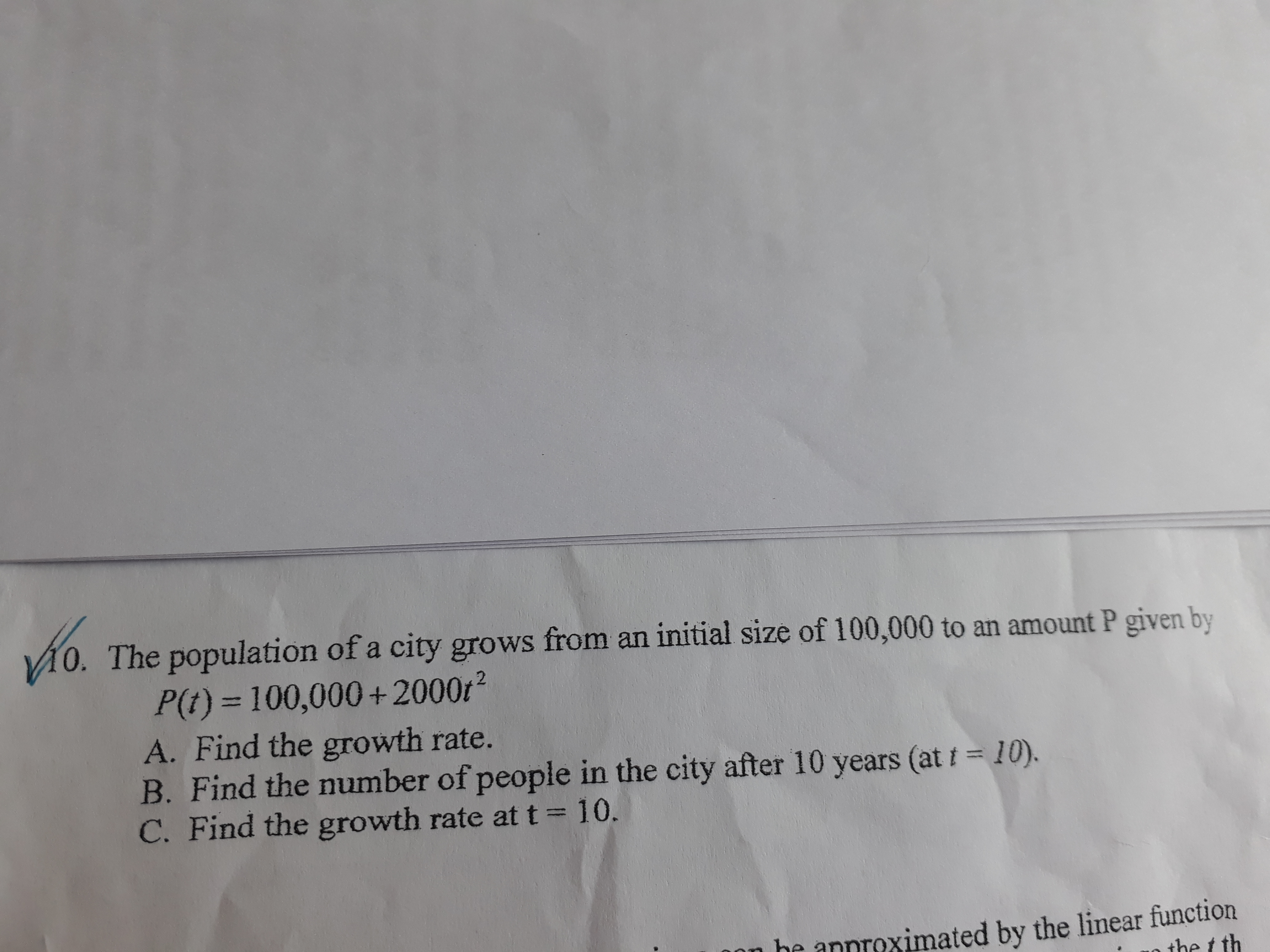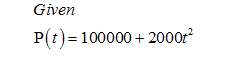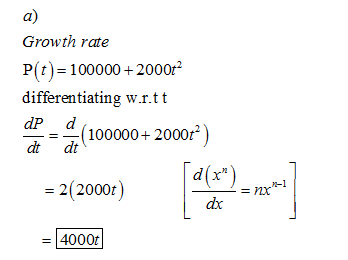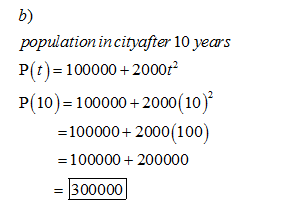# The population of a city grows from an initial size of 100,000 to an amount P given byP(t) = 100,000+2000r²A. Find the growth rate.B. Find the number of people in the city after 10 years (at t = 10).C. Find the growth rate at t = 10,he annroximated by the linear functionthe t th

Question
4 viewshelp_outlineImage TranscriptioncloseThe population of a city grows from an initial size of 100,000 to an amount P given by P(t) = 100,000+2000r² A. Find the growth rate. B. Find the number of people in the city after 10 years (at t = 10). C. Find the growth rate at t = 10, he annroximated by the linear function the t th fullscreen
check_circle

Step 1Step 2Step 3...

### Want to see the full answer?

See Solution

#### Want to see this answer and more?

Solutions are written by subject experts who are available 24/7. Questions are typically answered within 1 hour.*

See Solution
*Response times may vary by subject and question.
Tagged in